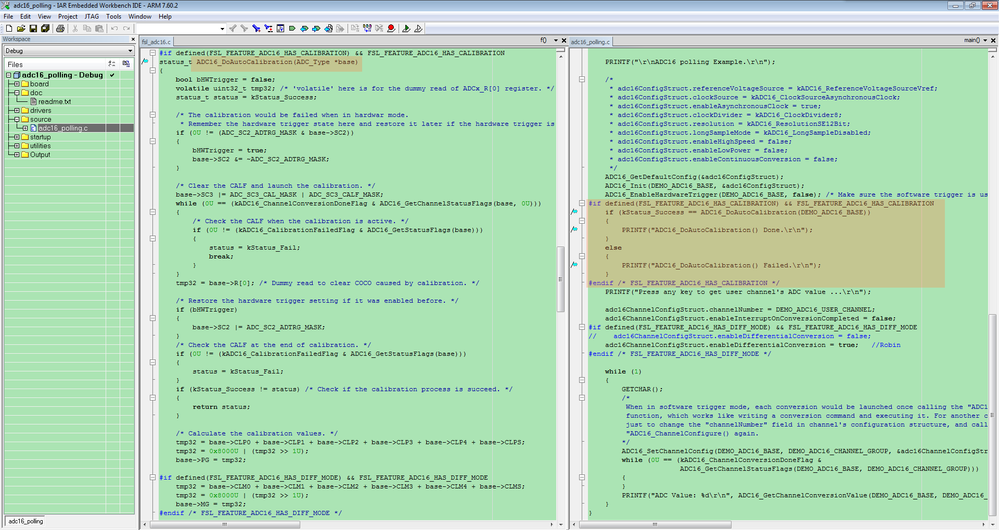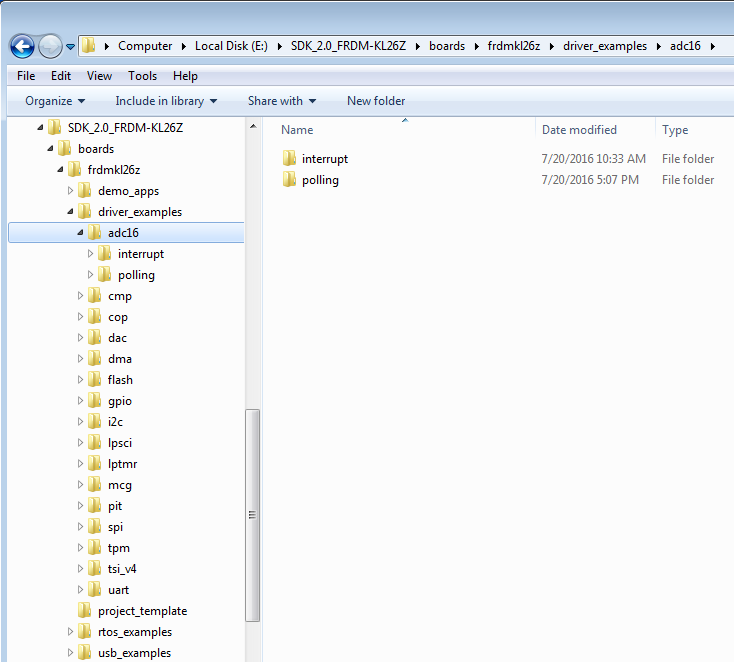cancel
Showing results for
Did you mean:
SOLVED

646 ViewsContributor I

I am attempting to configure the ADC on the FRDM-KL26Z in differential mode such that it takes a reading every time the read function is called in main().

I have an init() function:

```void adc_init(void){

and a calibration function that is called by the adc_init();

`void adc_cal(void){   //"for best calibration results" section is being ignored!!    //ADC0_SC2 = ADC_SC2_ADTRG(0x0);          //clear ADTRG bit    ADC0_SC3 = ADC_SC3_CAL_MASK;            //'user sets sc3[cal] and itll automatically begin'    /* Wait until calibration is finished */    while(ADC0->SC3 & ADC_SC3_CAL_MASK);    //calibrate high side    uint16_t cal_var_h = 0;                     //make a 16 bit var    cal_var_h = (ADC0_CLP0 & ~ADC_CLP0_CLP0_MASK) + (ADC0_CLP1 & ~ADC_CLP1_CLP1_MASK) + (ADC0_CLP2 & ~ADC_CLP2_CLP2_MASK)+ (ADC0_CLP3 & ~ADC_CLP3_CLP3_MASK) + (ADC0_CLP4 & ~ADC_CLP4_CLP4_MASK) + (ADC0_CLPS & ~ADC_CLPS_CLPS_MASK);           //sum all the calibrations    cal_var_h = cal_var_h / 2;              //divide the variable by two    cal_var_h = cal_var_h & 0x800;          //set the msb    ADC0_PG = cal_var_h;                    //store it where it belongs    //calibrate low side    uint16_t cal_var_l = 0;                     //make a 16 bit var    cal_var_l = (ADC0_CLM0 & ~ADC_CLM0_CLM0_MASK) + (ADC0_CLM1 & ~ADC_CLM1_CLM1_MASK) + (ADC0_CLM2 & ~ADC_CLM2_CLM2_MASK)+ (ADC0_CLM3 & ~ADC_CLM3_CLM3_MASK) + (ADC0_CLM4 & ~ADC_CLM4_CLM4_MASK) + (ADC0_CLMS & ~ADC_CLMS_CLMS_MASK);           //sum all the calibrations    cal_var_l = cal_var_l / 2;              //divide the variable by two    cal_var_l = cal_var_l & 0x800;          //set the msb    ADC0_MG = cal_var_l;                    //store it where it belongs    //ADC0_SC3 = ADC_SC3_CAL(0x0);            //disable calibration    if(ADC0_SC3 != 0)                       //check for success    {        uart_putc('f');                     //complain on the uart if calibration failed    }}`

`int16_t adc_get_read(uint8_t channel)    //signed int should handle the 2's compliment{    if(channel == 0)    {        //ADC0_CFG2 = ADC_CFG2_MUXSEL(0x0);        ADC0_SC1A |= ADC_SC1_ADCH(0x0);    //trigger conversion on ch0        while((ADC0_SC1A & ADC_SC1_COCO_MASK) == 0)  //conversion complete flag        {            //wait for the conversion to complete        }        //ADC0_SC1A = ADC_SC1_COCO(0x0);      //Reset COCO use the shift in        return ADC0_RA;                     //result register, should clear COCO    }/*nonfunctional channel 1 code snipped for brevity*/    else    //in case of burp    {        uart_putc('f');                     //complain on the uart if fail        return 0;    }}`

The function calls in main() look like

`reading0 = adc_get_read(0);uart_outHex(reading0);          //sends the reading over the UART to terminal in ascii encoded hex`

The frdm board is setup with R9 jumpered to enable external Vrefh, and Vrefh tied to 3.3V. DP0 is set to the output of the DAC and DM0 is set to ground.
The DAC, UART, and uart_outHex have been confirmed to work as expected and designed.

The failure is that the ADC reads very low values (0 through about 3, in hex) and very high values (0xFFFFFFFC through 0xFFFFFFFF) with no middle ground, and typically sticks to either high or low values with random deviations to the other value. I suspect that it is a clocking issue, but I can not determine where. Can anyone advise me? Thank you.

Labels (3)

• ### Kinetis M Series MCUs

Tags (4)
1 Solution
109 ViewsContributor I

cal_var_h and cal_var_l are calculated incorrectly- should just be a sum, and 0x800 should be 0x8000.

2 Replies
110 ViewsContributor I

cal_var_h and cal_var_l are calculated incorrectly- should just be a sum, and 0x800 should be 0x8000.

109 ViewsNXP TechSupport

Hi Karen,

You can refer the ADC16_DoAutoCalibration function in KSDK2.0 for KL26.I recommand you use the examples in KSDK2.0. (If you have not used it, please read Introducing Kinetis SDK v2 first.)You can download and install it refer the methods in How to: install KSDK 2.0.

Best Regards,

Robin